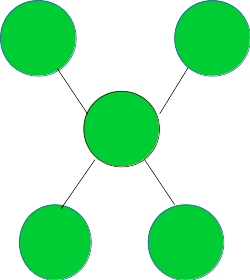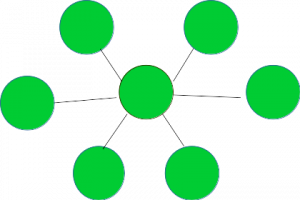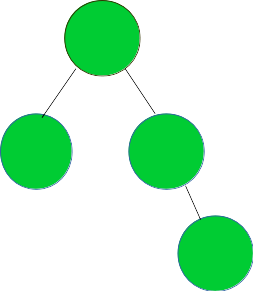# Check if the given graph represents a Star Topology

Given a graph G, the task is to check if it represents a Star Topology.

A Star Topology is the one shown in the image below:Examples:

```Input : Graph =Output : YES

Input : Graph =Output : NO
```

## Recommended: Please try your approach on {IDE} first, before moving on to the solution.

A graph of V vertices represents a star topology if it satisfies the following three conditions:

1. One node (also called the central node) has degree V – 1.
2. All nodes except the central node have degree 1.
3. No of edges = No of Vertices – 1.

The idea is to traverse the graph and check if it satisfies the above three conditions. If yes, then it represents a Star Topology.

Below is the implementation of the above approach:

## CPP

 `// CPP program to check if the given graph ` `// represents a Star Topology ` `#include ` ` `  `using` `namespace` `std; ` ` `  `// A utility function to add an edge in an ` `// undirected graph. ` `void` `addEdge(vector<``int``> adj[], ``int` `u, ``int` `v) ` `{ ` `    ``adj[u].push_back(v); ` `    ``adj[v].push_back(u); ` `} ` ` `  `// A utility function to print the adjacency list ` `// representation of graph ` `void` `printGraph(vector<``int``> adj[], ``int` `V) ` `{ ` `    ``for` `(``int` `v = 0; v < V; ++v) { ` `        ``cout << ``"\n Adjacency list of vertex "` `             ``<< v << ``"\n head "``; ` `        ``for` `(``auto` `x : adj[v]) ` `            ``cout << ``"-> "` `<< x; ` `        ``printf``(``"\n"``); ` `    ``} ` `} ` ` `  `/* Function to return true if the graph represented  ` `   ``by the adjacency list represents a Star topology  ` `   ``else return false */` `bool` `checkStarTopologyUtil(vector<``int``> adj[], ``int` `V, ``int` `E) ` `{ ` ` `  `    ``// Number of edges should be equal ` `    ``// to (Number of vertices - 1) ` `    ``if` `(E != (V - 1)) ` `        ``return` `false``; ` ` `  `    ``// a single node is termed as a star topology ` `    ``// having only a central node ` `    ``if` `(V == 1) ` `        ``return` `true``; ` ` `  `    ``int``* vertexDegree = ``new` `int``[V + 1]; ` `    ``memset``(vertexDegree, 0, ``sizeof` `vertexDegree); ` ` `  `    ``// calculate the degree of each vertex ` `    ``for` `(``int` `i = 1; i <= V; i++) { ` `        ``for` `(``auto` `v : adj[i]) { ` `            ``vertexDegree[v]++; ` `        ``} ` `    ``} ` ` `  `    ``// countCentralNodes strores the count of nodes  ` `    ``// with degree V - 1, which should be equal to 1 ` `    ``// in case of star topology ` `    ``int` `countCentralNodes = 0, centralNode = 0; ` ` `  `    ``for` `(``int` `i = 1; i <= V; i++) { ` `        ``if` `(vertexDegree[i] == (V - 1)) { ` `            ``countCentralNodes++; ` `            ``// Store the index of the central node ` `            ``centralNode = i; ` `        ``} ` `    ``} ` ` `  `    ``// there should be only one central node ` `    ``// in the star topology ` `    ``if` `(countCentralNodes != 1) ` `        ``return` `false``; ` ` `  `    ``for` `(``int` `i = 1; i <= V; i++) { ` `        ``// except for the central node  ` `        ``// check if all other nodes have ` `        ``// degree 1, if not return false ` `        ``if` `(i == centralNode) ` `            ``continue``; ` `        ``if` `(vertexDegree[i] != 1) { ` `            ``return` `false``; ` `        ``} ` `    ``} ` ` `  `    ``// if all three necessary ` `    ``// conditions as discussed, ` `    ``// satisfy return true ` `    ``return` `true``; ` `} ` ` `  `// Function to check if the graph  ` `// represents a Star topology ` `void` `checkStarTopology(vector<``int``> adj[], ``int` `V, ``int` `E) ` `{ ` `    ``bool` `isStar = checkStarTopologyUtil(adj, V, E); ` `    ``if` `(isStar) { ` `        ``cout << ``"YES"` `<< endl; ` `    ``} ` `    ``else` `{ ` `        ``cout << ``"NO"` `<< endl; ` `    ``} ` `} ` ` `  `// Driver code ` `int` `main() ` `{ ` `    ``// Graph 1 ` `    ``int` `V = 5, E = 4; ` `    ``vector<``int``> adj1[V + 1]; ` `    ``addEdge(adj1, 1, 2); ` `    ``addEdge(adj1, 1, 3); ` `    ``addEdge(adj1, 1, 4); ` `    ``addEdge(adj1, 1, 5); ` `    ``checkStarTopology(adj1, V, E); ` ` `  `    ``// Graph 2 ` `    ``V = 5, E = 4; ` `    ``vector<``int``> adj2[V + 1]; ` `    ``addEdge(adj2, 1, 2); ` `    ``addEdge(adj2, 1, 3); ` `    ``addEdge(adj2, 3, 4); ` `    ``addEdge(adj2, 4, 5); ` `    ``checkStarTopology(adj2, V, E); ` ` `  `    ``return` `0; ` `} `

## Python3

 `# Python3 program to check if the given graph ` `# represents a star topology ` ` `  `# A utility function to add an edge in an ` `# undirected graph. ` `def` `addEdge(adj, u, v): ` `    ``adj[u].append(v) ` `    ``adj[v].append(u) ` ` `  `# A utility function to prthe adjacency list ` `# representation of graph ` `def` `printGraph(adj, V): ` ` `  `    ``for` `v ``in` `range``(V): ` `        ``print``(``"Adjacency list of vertex "``,v,``"\n head "``) ` `        ``for` `x ``in` `adj[v]: ` `            ``print``(``"-> "``,x,end``=``" "``) ` `        ``printf() ` ` `  `# /* Function to return true if the graph represented ` `#    by the adjacency list represents a star topology ` `#    else return false */ ` `def` `checkStarTopologyUtil(adj, V, E): ` ` `  `    ``# Number of edges should be equal ` `    ``# to (Number of vertices - 1) ` `    ``if` `(E !``=` `(V ``-` `1``)): ` `        ``return` `False` ` `  `    ``# a single node is termed as a bus topology ` `    ``if` `(V ``=``=` `1``): ` `        ``return` `True` ` `  `    ``vertexDegree ``=` `[``0``]``*``(V ``+` `1``) ` ` `  `    ``# calculate the degree of each vertex ` `    ``for` `i ``in` `range``(V``+``1``): ` `        ``for` `v ``in` `adj[i]: ` `            ``vertexDegree[v] ``+``=` `1` ` `  `    ``# countCentralNodes strores the count of nodes ` `    ``# with degree V - 1, which should be equal to 1 ` `    ``# in case of star topology ` `    ``countCentralNodes ``=` `0` `    ``centralNode ``=` `0` ` `  `    ``for` `i ``in` `range``(``1``, V ``+` `1``): ` `        ``if` `(vertexDegree[i] ``=``=` `(V ``-` `1``)): ` `            ``countCentralNodes ``+``=` `1` `            ``# Store the index of the central node ` `            ``centralNode ``=` `i ` ` `  `    ``# there should be only one central node ` `    ``# in the star topology ` `    ``if` `(countCentralNodes !``=` `1``): ` `        ``return` `False` ` `  `    ``for` `i ``in` `range``(``1``, V ``+` `1``): ` `        ``# except for the central node ` `        ``# check if all other nodes have ` `        ``# degree 1, if not return false ` `        ``if` `(i ``=``=` `centralNode): ` `            ``continue` `        ``if` `(vertexDegree[i] !``=` `1``): ` `            ``return` `False` ` `  `    ``# if all three necessary ` `    ``# conditions as discussed, ` `    ``# satisfy return true ` `    ``return` `True` ` `  `# Function to check if the graph represents a bus topology ` `def` `checkStarTopology(adj, V, E): ` ` `  `    ``isStar ``=` `checkStarTopologyUtil(adj, V, E) ` `    ``if` `(isStar): ` `        ``print``(``"YES"``) ` ` `  `    ``else``: ` `        ``print``(``"NO"` `) ` ` `  `# Driver code ` ` `  `# Graph 1 ` `V, E ``=` `5``, ``4` `adj1``=``[[] ``for` `i ``in` `range``(V ``+` `1``)] ` `addEdge(adj1, ``1``, ``2``) ` `addEdge(adj1, ``1``, ``3``) ` `addEdge(adj1, ``1``, ``4``) ` `addEdge(adj1, ``1``, ``5``) ` `checkStarTopology(adj1, V, E) ` ` `  `# Graph 2 ` `V, E ``=` `4``, ``4` `adj2``=``[[] ``for` `i ``in` `range``(V ``+` `1``)] ` `addEdge(adj2, ``1``, ``2``) ` `addEdge(adj2, ``1``, ``3``) ` `addEdge(adj2, ``3``, ``4``) ` `addEdge(adj2, ``4``, ``2``) ` `checkStarTopology(adj2, V, E) ` ` `  `# This code is contribued by mohit kumar 29 `

Output:

```YES
NO
```

Time Complexity: O(V + E) where V and E are the numbers of vertices and edges in the graph respectively.My Personal Notes arrow_drop_upCheck out this Author's contributed articles.

If you like GeeksforGeeks and would like to contribute, you can also write an article using contribute.geeksforgeeks.org or mail your article to contribute@geeksforgeeks.org. See your article appearing on the GeeksforGeeks main page and help other Geeks.

Please Improve this article if you find anything incorrect by clicking on the "Improve Article" button below.

Improved By : mohit kumar 29